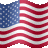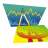# Leavitt Projection Indicator for ThinkorSwim#### J007RMC

##### Active member
2019 Donor
VIP
Im using this its a moving average similar to the hull although I like this better it very responsive to price change.
Code:
``````# Leavitt, Jay A., PhD . “Beyond The Hull With Leavitt Projections,” Technical Analysis of StockS & commoditieS, Volume 35: February.

script LeavittProjection{
input y = close;
input n = 20;
rec x = x + 1;
def a = (n * sum(x * y, n) - sum(x, n) * sum(y, n) ) / ( n *sum(Sqr(x), n) - Sqr(sum(x, n)));
def b = (sum(Sqr(x), n) * sum(y, n) - sum(x, n) * sum(x *y, n) ) / ( n * sum(Sqr(x), n) - Sqr(sum(x, n)));
plot LeavittProjection= a*x+ b;
}
script LeavittConvolution
{ input price = close;
input n = 20;
def intLength = Floor(Sqrt(n));
plot LeavittConvolution = LeavittProjection (LeavittProjection (price, n), intLength);
}
def price = Close;
input length = 9;
def intLength = Floor(Sqrt(length));
plot LeavittConvolution = LeavittProjection (LeavittProjection (price, length), intLength);
LeavittConvolution.AssignValueColor(if LeavittConvolution > LeavittConvolution  then Color.GREEN else Color.RED);``````#### horserider

##### Well-known member
VIP
Looked at this a while ago. Decided a SMA of 3 or just the regular candle colors worked better. Then tried different lengths and still could not make it useful for me. Maybe you can display the way you trade this. Always willing to revisit it if can be proven useful.#### J007RMC

##### Active member
2019 Donor
VIP
Using the 3min as the signal of change I can follow the indicator up so far on the 5min and usually all the way up on the 15min or down so I warch the 3,5 and 15min for the fluidity of change.#### J007RMC

##### Active member
2019 Donor
VIP
I'm also using the zero lag Bollinger band as a lower so I watch both indicators
Code:
``````# Zero Lag MACD With Bollinger Bands
# Mobius
# V01.01.2018 Chat Room Request .0.19.2018

declare lower;

input fastLength = 13;
input slowLength = 34;
input MACDLength = 13;
input showBreakoutSignals = yes;

script EMA{
input length = 10;
input price = close;
def K = 2 / (length + 1);
def lag = (length - 1) / 2;
def ZLEMA = K * (2 * price - price[lag]) + (1 - K) * ZLEMA;
plot data = ZLEMA;
}
plot Value = EMA(length = fastLength) - EMA(length = slowLength);
def Avg = EMA(length = MACDLength, price = value);
plot Diff = Value - Avg;
def SD = StDev(Diff, MACDLength);
plot upper = Diff + SD;
plot lower = Diff - SD;
plot ZeroLine = 0;
Value.SetPaintingStrategy(PaintingStrategy.Line_Vs_Points);
Value.AssignValueColor(if Value > Value
then color.green
else color.red);
Diff.SetStyle(Curve.Short_Dash);
Diff.SetLineWeight(1);
Diff.SetDefaultColor(color.gray);
upper.SetDefaultColor(color.gray);
lower.SetDefaultColor(color.gray);
ZeroLine.SetDefaultColor(GetColor(0));
ZeroLine.HideBubble();
ZeroLine.HideTitle();

# End Code Zero Lag MACD``````

•mashume#### J007RMC

##### Active member
2019 Donor
VIP#### horserider

##### Well-known member
VIP
Not getting that ZLBB. Think the Ultimate MACD may be more useful. Check out if it works for your plan.#### J007RMC

##### Active member
2019 Donor
VIP
Ill put that on the chart for tomorrow many thanks....#### J007RMC

##### Active member
2019 Donor
VIP
Horserider I like the ultimate macd So to trade it above and below the zero and or bb midline?#### horserider

##### Well-known member
VIP
@J007RMC So many ways to trade it. Easiest and fastest would be change of MACD colors. The white line is the exp avg of MACD value. So can trade that cross. Blue is the midline of MACD BB can trade that cross. Both can be used as confirmations you are still going in the same direction. For fast trades on 3 min if BBlength is set to 5 That would be first cross. If BBlength is set to 20. The AVG would be first cross. Remember the BBmidline is like a moving average of price. Confused yet ? With the MACD bands acting as Bollinger Bands you can see volatility. With the MACD being inside the bands you can now trade the MACD with the same rules as price and Bollinger bands. So the best setup would be a squeeze of the bands and then getting a breakout with the MACD riding a band up or down. Then the first hint of weakening of that move would be the separation of the MACD from the band. As far as the zero line it can be seen as an indication of the strength or weakness of the MACD, below zero = weaker and above zero = strength. It is extrememly configurable to fit your trading style. Hope that helps.

•mashume and diazlaz#### J007RMC

##### Active member
2019 Donor
VIP
Oh my gosh yes this helps a bunch thanks

R

#### Rukkit

##### New member
VIP
Anyway to make this indicator show up properly on mobile app?Time Series Projection Trend for ThinkorSwim Indicators 8Easycator's VWAP Trading Indicator/Strategy Questions 8